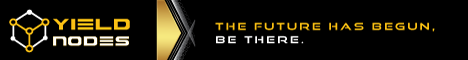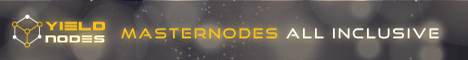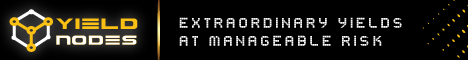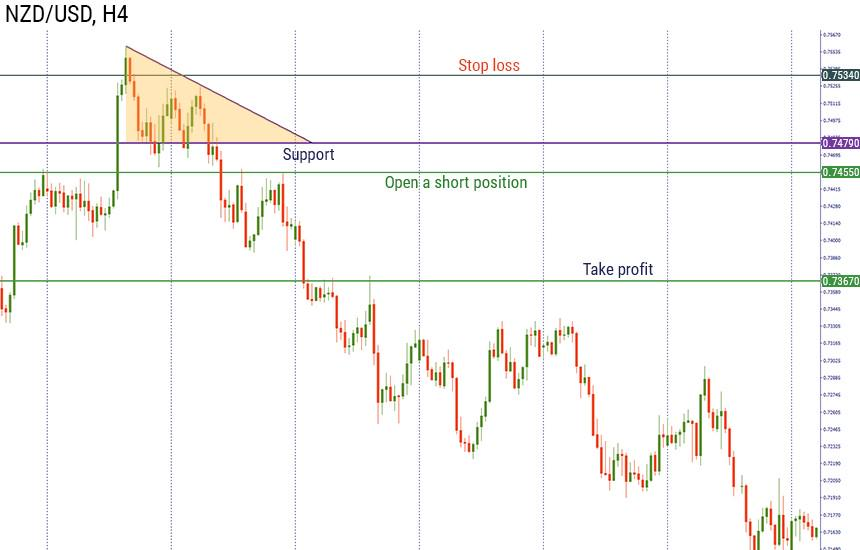# Triangle Pattern and How to Use it in Forex?

0
781
views

Have you ever counted stars while looking for a constellation? Or maybe you imagined yourself as an astrologer, trying to predict the future?

In trading, you can do the same by looking at the charts and patterns. Moreover, if your analysis is correct, your future-telling may help you to earn money.   In this article, we are going to present you the simple strategies for using one of the famous and easy recognizable patterns. We are talking about the triangle pattern.

## Introduction to the triangle chart pattern

Triangle patterns are constructed by straight lines of highs and lows. In most of the situations, this is a continuation chart pattern that is why you need to pay specific attention to the price action.

As you know from the Forex Guidebook, there are three types of triangle chart patterns:

• Ascending triangle pattern;
• Descending triangle pattern;
• Symmetrical triangle pattern.

The main difference between them lies in their slope. The ascending triangle pattern shows that bulls are getting stronger. As a result, its slope goes up.  In its turn, the descending triangle pattern demonstrates the strength of bears.'; \$bannerAd = ''; \$bannerAd = ''; \$adCount = count(\$bannerAd); \$randomAdNumber = mt_rand(1, \$adCount); echo \$bannerAd[\$randomAdNumber]; ?>

The most interesting situation is connected with the symmetrical triangle. The price action in this chart pattern shows an equal balance between bulls and bears. That is, the breakout of the pattern may be either to the upside or the downside.

## Trading strategies for different types of triangle pattern

At first, let’s consider the situation when a symmetrical triangle occurs.  On the daily chart of EURUSD, the intersection of a rising trendline and a downward line within a large downtrend formed the desirable pattern. After the pattern was implemented, we waited for the breakout to the downside, or to the upside. In our example, the candlestick broke the upper border of the triangle, as a result, we entered the position at 1.3355. We placed stop loss about 10 pips below the lowest point of the triangle. That is, we placed our stop loss at 1.2324.  Our take profit equals to the height of the triangle. We measure the destination between the highest and the lowest points of the pattern:

1.3294-1.2334=0.096.

So, we place our take profit at 1.4315 (1.3355+0.096=1.4315)Now let’s find out how to trade the ascending triangle. Traders always think about this pattern as of bullish one, which is characterized by breaking out above resistance when the pattern is implemented. However, it doesn’t happen all the time. The breakout of the lower border of the ascending triangle may happen during the downtrend.

Let’s consider the situation when the ascending triangle is implemented within the uptrend.

On the H4 chart of NZDUSD, the ascending pattern was formed between the upper border at the resistance at 0.7314 and the lower border formed by the uptrend. We decided to open the position after the break of the resistance at 0.7333. We place a stop loss at 0.7225 (10 pips below the lowest point of the triangle) and our take profit equals 0.7413 (highest point at 0.7314 – the lowest point at 0.7234= 0.08)

0.7333+0.08=0.7413Finally, let’s look at how to trade the descending triangle. It can be described by strong support intersecting a downtrend line. If this pattern occurs during the downtrend, this will signal a continuation of this movement.

On the H4, the consolidation at the top of the upward trend shows bulls unable to push the price higher. As a result, the descending triangle was implemented with the support at 0.7470. We decided to enter the market on the breakout of the support, so we place a short order at 0.7455.
Our stop loss is placed 10 pips above the last swing high at 0.7534 and take profit is placed at 0.7367.### Conclusion

If you know how triangles are implemented, this may provide you a good opportunity to boost your profit. But be careful! Triangles may trick your mind if they move oppositely to the trend. That is why you need to be sure about the correct implementation and in the market conditions.'; \$bannerAd = ''; \$bannerAd = ''; \$adCount = count(\$bannerAd); \$randomAdNumber = mt_rand(1, \$adCount); echo \$bannerAd[\$randomAdNumber]; ?>